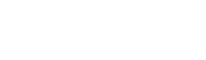# Solving FizzBuzz Problems Using Basic Python Grammar

スポンサーリンク

Hello, I’m Yuki, a student engineer.

If you want to become an engineer, you probably see FizzBuzz problems in entry-level tests or intern coding tests.

I think FizzBuzz problems are a great way to learn programming thinking, so I’m going to write an article about them.

スポンサーリンク

## What is FizzBuzz proplem?

The FizzBuzz problem was proposed by Jeff Atwood, co-owner of Stack Overflow.

It is often used to identify whether an aspiring programmer can code.

The FizzBuzz problem is here.

Write programming to output numbers from 1 to 100.

However, when the number is a multiple of 3, output Fizz instead of the number, when the number is a multiple of 5, output Buzz instead of the number, and when the number is a multiple of 3 and 5, output FizzBuzz.

## How to implement the code

The algorithm for the FizzBuzz questions is as follows.

Multiples of 3 and multiples of 5 → FizzBuzz

Multiple of 3 → Fizz

Multiples of 5 → Buzz

Other multiples→Output a number

## Coding

Here is the implementation code

for i in range(1,101):
if i % 3 == 0 and i % 5 == 0:
print("FizzBuzz")
elif i % 3 == 0:
print("Fizz")
elif i % 5 == 0:
print("Buzz")
else:
print(i)

Output:

1
2
Fizz
4
Buzz
Fizz
7
8
Fizz
Buzz
11
Fizz
13
14
FizzBuzz

## At the end

Many programmers seem to be solving FizzBuzz problems with restrictions such as how short the code should be, how it should be implemented without using the “%” sign, and so on.

If you can solve FizzBuzz problems, you are on your way to becoming a great programmer.

Yuki